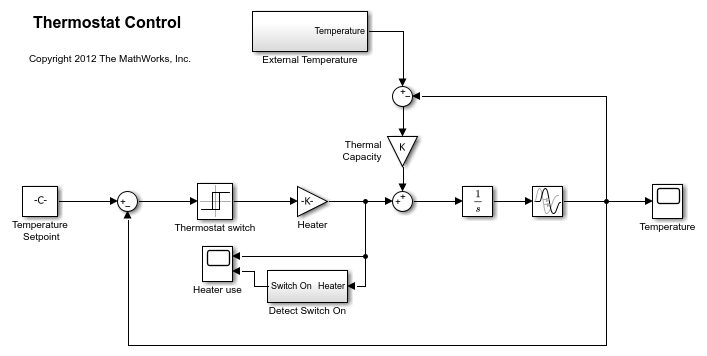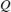# Skip Model Simulation Based on Parameter Constraint Violation (GUI)

This example shows how to optimize a design and specify parameter-only constraints that prevent the model from being evaluated in an invalid solution space.

During optimization, the solver may try a design variable set that results in a model simulation error, which can be computationally expensive. If you can define a parameter-only constraint that identifies such a design variable set, then the solver can use the constraint to skip such sets. In other words, you can configure the optimization to be more efficient by disallowing design variable sets that lead to simulation errors.

In this example, you optimize thermostat settings to minimize temperature set-point deviations while satisfying some constraints. One of the constraints applies to the model parameters that define the thermostat switch on/switch off points. If the switch-off point is greater than the switch-on point, evaluating the model leads to a simulation error.

### Thermostat Model

Open the model.

open_system('sdoThermostat');The model describes a simple heater & thermostat that regulate the temperature of a room. The room is subject to external temperature fluctuations. The room temperature is computed using a first-order heat-flow equation:Where:

•is the room temperature (C).

•the external temperature (C).

•the heat supplied by the heater (W).

•the room thermal capacity (J/C).

The heater is controlled by a thermostat that turns on when the difference between the room temperature and temperature set-point exceeds a threshold. The heater turns off when the error drops below a threshold.

### Thermostat Design Problem

You tune the thermostat turn-on and turn-off temperature thresholds, and also the heater power. The Thermostat switch block specifies the turn-on and turn-off thresholds using the variables H_on and H_off. The Heater block specifies the heater power using the variable Hgain.

The design requirements are:

• Minimize the difference between the room temperature and temperature set-point over a 24 hour period.

• The heater must not turn on more than 12 times during the 24 hour period.

• The thermostat turn-on temperature must be greater than the thermostat turn-off temperature. If this constraint is violated, the model is invalid and cannot be simulated or evaluated.

### Open the Response Optimizer

Open a pre-configured Response Optimizer session using the following commands.

load sdoThermostat_sdosession sdotool(SDOSessionData)The pre-configured session specifies the following variables:

• DesignVars - Design variables set for the H_on, H_off, and Hgain model parameters.

• Minimize_T_error - Requirement to minimize the temperature deviation from the set-point.

• LimitH_on - Requirement to limit the number of times the thermostat is turned on.

• H_on_sig and T_error - Logged signals. H_on_sig represents when the heater is on. T_error is the difference between the room temperature and the set-point.

### Specify Parameter Constraint

The H_on > H_off requirement is not yet defined. Use a custom requirement to specify this constraint and configure the requirement to error if it is not satisfied.

In the New drop-down list, select Custom Requirement. The Create Requirement dialog opens.

In this dialog, specify the following:

• Name - SwitchConstraint.

• Type - Select Constrain the function output to be >= 0 from the Type list.

• Function - @sdoThermostat_SwitchingConstraint.

• Error if constraint is violated - Select this check box.The software calls the sdoThermostat_SwitchingConstraint function at each optimization iteration with a structure containing all the design variables. The output of the sdoThermostat_SwitchingConstraint function is the difference between the H_on and H_off values. This difference must be positive for the requirement to be satisfied.

The software evaluates custom requirements that test parameter-only constraints, such as SwitchConstraint, before simulating the model and evaluating the remaining requirements.

• If the constraint is violated while the Error if constraint is violated check box is selected, the software does not simulate the model to evaluate the remaining requirements. Instead, the solver assigns the cost function a NaN value for this iteration, evaluates the terminating conditions, and continues.

• If the constraint is violated while the Error if constraint is violated check box is cleared, the solver will attempt to simulate the model to evaluate the remaining requirements. Simulating the model may lead to a hard error; for example, simulating the thermostat model when SwitchConstraint is violated will lead to an error. In this case, the solver assigns the cost function a NaN value for this iteration, evaluates the terminating conditions, and continues.

To examine the constraint function, type edit sdoThermostat_SwitchingConstraint. The requirement that H_on > H_off is implemented as H_on - H_off > 0

### Optimize the Design

Click Optimize.The Optimization Progress window appears and updates at each iteration. The optimization successfully minimizes the temperature error while satisfying the switching constraints.

During this optimization, the H_on and H_off values never approach the H_on > H_off constraint boundary. So, there is never a danger of violating the constraint. However, changing the optimization algorithm may produce different behavior. For example, changing the optimization algorithm from the one used here, 'Interior-Point', to 'Active-Set' results in H_on and H_off values that are at the constraint boundary. This violation triggers the SwitchConstraint requirement and prevents model simulation for the relevant iterations.

### View Optimized Model Response

Simulate the model with the optimized thermostat settings. The optimized heater operation is displayed in the Heater use scope where the upper axis is the delivered heat and the lower axis the heater switch on times.The optimized room temperature is displayed in the Temperature scope.Close the model.

bdclose('sdoThermostat')